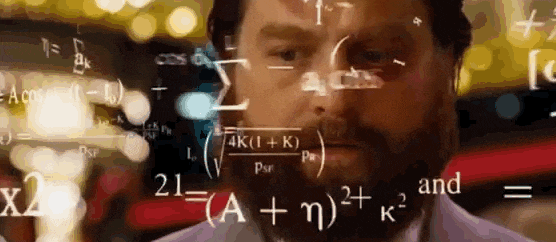# Maths! Trying to learn it (rawr)

So, long story short, I neglected to learn a large portion of mathematics growing up. Though now I am trying to learn it, I generally had one question that I am hoping someone is kind enough to clarify for me?

Whenever an equation is written, (a long one), people generally have brackets being used.
Example:

56 x 3 ( 7[4 x 8] ) - 3 + ( 4 x 4 [ 5 -3 ] ) = …

I don’t actually even know if that even makes sense. But, as a non-mathematician, it’s what these long equations look like to me.
I also see people use * , is that just another way to use x as multiplication?

And what do we call this? Is this stuff algebra?

I just feel that if I can learn this stuff, a lot more of the maths-sided EVE stuff would make a lot more sense to me; because right now, whenever I read calculations etc made in the forums …I am lost. But I want to understand.

Thank you for taking the time to help!

2 Likes

When they tryina figure out how I caught their travel ceptor…2 Likes

Good on you for trying to learn!

Yes, `*` is used as an alternative symbol for `x` denoting multiplication. This is usually done because in algebra, you often have a variable `x` to solve for, let’s say “`2x+2=4`, what is the value of `x`?” Sometimes, as in the equation before, you omit the multiplication symbol when it is between a number and a variable (so `2x` means `2*x`).

The brackets are there to denote the priority of operations. Normally, you do multiplications before additions, so `2*3+1` is `2*3=6` then `6+1=7`, however, `2*(3+1)` is `3+1=4` then `2*4=8` so it does make a difference.

Algebra is more solving equations for the value of a variable, like the above `2x+2=4, x=?`, or more complicated ones with multiple different variables. Adding, subtracting, multiplying and dividing numbers is arithmetic.

1 Like

Scalar multiplication can be written in several ways:

• informally, the multiplication sign `×` is most common, as in `2×4 = 8` or `2×a = 8`.
• in computer code, the asterisk `*` has taken over from the multiplication sign, because the latter wasn’t included in the ASCII character set, and to avoid confusion with the letter `x`. So, in code that becomes `2*4 = 8` or `2*a = 8` (though neither of those are proper assignments, but that is out of scope here)
• in algebra, the dot operator `⋅` is used to differentiate between the multiplication sign `×`, the letter `x`, and the cross product `⨯` (which is used for vector and matrix multiplication). The above product is then written as `2⋅4 = 8` or `2⋅a = 8`.
• also common in algebra is to omit the multiplication operator altogether, but only if it wouldn’t cause any ambiguity. So, `2a = 8` is ok, but `24 = 8` is not (obviously). This is most common between variables, and between brackets. e.g. `2ab(4b+6)(2b+1)` actually means (when written with the multiplication sign) `2×a×b×(4×b+6)×(2×b+1)`.

As for operation grouping through brackets, the most commonly-used symbols for that are the parentheses `(` and `)`. They alter the default operator precedence rules, in which exponents (e.g. `a³`) are applied first, then factors (e.g. `3×a`), and finally terms (e.g. `a+3`). Operations between brackets are always done first, with their result taking the place of the contents of the brackets. When only a single variable or a constant remains between the brackets, or when the brackets are the outer part of the formula, they can be safely omitted. (i.e. `(2) = 2`, `(a) = a`, and `(2b+4c+6) = 2b+4c+6`).

Note the difference between

• `2+3×4² = 2+3×16 = 2+48 = 50`
• `2+(3×4)² = 2+(12)² = 2+12² = 2+144 = 146`
• `(2+3)×4² = (5)×4² = 5×4² = 5×16 = 80`
• `(2+3×4)² = (2+12)² = (14)² = 14² = 196`

The use of square brackets `[` `]` is more commonly used for matrix notation, while angle brackets `⟨` `⟩` are used to denote the inner product of vectors. But there’s a lot of variation here, with parentheses being used for matrix and vector notiation, and the dot operator for the inner product of vectors in euclidean space.

1 Like

Wow! Thank you both for the detailed responses.
I’m not gonna lie, some of the terminology used was slightly over-head, but the information I sought clarified was indeed clarified.
Formula are beginning to make sense.

And thus my mathematical journey continues …

I appreciate the help.

5 Likes

Take a look at this. They have excellent lectures and you can learn at your own pace:

Good for you. However, the forums kinda suck in terms of mathematics notation (that is there are limitations that make things a bit harder than if we were using paper or a blackboard). However, in addition to the responses others have made consider the order of operations as well.

This can trip people up in that they’ll do the mathematical operations out of order and get the wrong answer.

See! Maths can be fun!
Everything is a function of one
And learning really isnt hard
When you’ve got your library card

1 Like

This topic was automatically closed 90 days after the last reply. New replies are no longer allowed.